International Journal of Geomagnetism and Aeronomy
Vol. 3, No. 2, December 2002

# Coherent nonlinear interaction of waves in collisional ionospheric plasma

A. V. Volosevich

Mogilev State University, Mogilev, Belarus

C.-V. Meister

Astrophysical Institute, Potsdam, Germany

### Abstract

In this paper, the coherent nonlinear interaction of three and four electrostatic Farley-Buneman waves is considered analytically and numerically. The evolution of the nonlinear waves is described by a system of magnetohydrodynamic (MHD) equations. It is shown that the interaction of the three or four coherent waves is the main physical mechanism that leads to the saturation of the Farley-Buneman instability. Through the interaction of the coherent waves, nonlinear waves and nonlinear structures are generated when the waves are damped linearly and propagate perpendicular to the electron drift velocity. This wave region corresponds to large aspects and flow angles of the small-scale waves. Further, the wave interaction causes a nonlinear stabilization of the growth of the high-frequency waves. Density modifications of the charged particles during the nonlinear stage of wave growth are also estimated.

### 1. Introduction

The auroral radio scatter experiments EISCAT and STARE reveal that in the E region of the auroral ionosphere, rather intensive electrostatic structures form, that are connected with an essential modification of the density distribution of the charged background particles. According to a widespread point of view, these plasma structures can be a consequence of the excitation of the modified two-stream or Farley-Buneman (FB) plasma turbulence. The linear theory of the FB instability effectively explains many of the observed phenomena of radar echoes, including the conditions necessary for the onset of the FB waves. But in spite of the theoretical and experimental research successes in auroral radar scattering, many problems yet exist in understanding the experimental data.

A central question of the research of the irregularities in the auroral E region ionosphere are the echoes at large angles with respect to the electron drift velocity in the plane perpendicular to the geomagnetic field ("large flow angle") and the echoes at a few degrees off the perpendicular plane ("large aspect angle"). The theoretical explanations of these effects are very difficult and controversial. Attempts to solve the problems were made by considering refraction effects [Uspensky et al., 1994], effects due to strong currents and anomalous resistivity [Hamza and St. Maurice, 1995; Volosevich and Liperovsky, 1975], resonance broadening [Robinson and Honary, 1990; Sudan, 1983], and mode-coupling [Otani and Oppenheim, 1998; Schlegel and Thiemann, 1994].

Many efforts have already been made to investigate the nonlinear evolution of FB waves analytically [Hamza and St. Maurice, 1993; Sahr and Fejer, 1996; Sudan, 1983] and by computer simulations [Fedorov, 1988; Janhunen, 1994; Newman and Ott, 1981; Oppenheim and Otani, 1996; Schlegel and Thiemann, 1994].

In this paper, the coherent nonlinear interaction of three and four FB waves is considered analytically and numerically. The evolution of the nonlinear waves is described by a system of connected MHD equations for the amplitudes of the interacting waves.

### 2. Theoretical Model

According to classical linear theory, in collisional plasmas with magnetized electrons, newce, and non-magnetized ions, niwci, ( ne, ni are the collision frequencies of the electrons and the ions with the neutral particles; wce, wci designate the electron and ion gyrofrequencies), the FB instability may occur in the presence of electrostatic fields. The dispersion equation of linearly growing waves with frequency w<ne,ni reads [Volosevich and Galperin, 1997]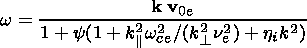(1)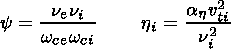hi is the ion dynamical viscosity, a<FONT FACE='Symbol'>h designates a dimensionless constant depending on the type of collisions in the plasma (within theory - depending on the type of chosen collision integral), k and k<IMG SRC='latex083.gif' ALT="bot"> are the components of the wave vector k parallel and perpendicular to the magnetic field B=B0 ez, v0e is the electron drift velocity, and vti=(2 Ti/mi)1/2 is the thermal velocity of the ions. Ti represents the ion temperature in Boltzmann constants kB, and mi is the ion mass.

From the linear theory, one can conclude that the condition for the frequency of the FB waves w<ni is not satisfied if the irregularities have scales L<2pv0e/ni (at altitudes of h100 km above the surface of the Earth, at which ni2103 s -1 and v0e6102 m s -1, it follows L<2 m). Besides, in the upper E region, plasma conditions with w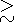ne are also possible. In the works [Lee et al., 1971; Schlegel and Thiemann, 1994], it was found that linearly growing wave modes excited by electron-neutral collisions have a frequency w<wc, w2c=wcewci.

Further, considering the action of neutral winds in the E region, it was shown that the dispersion relation of linear FB waves has three solutions [Liperovsky et al., 1996; Meister, 1995]. Two wave modes are damped, and the third mode which has frequencies about one order smaller than the frequencies of the damped waves, is linearly unstable. The unstable mode has wavelengths k of about 1/m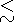k70/ m, and the maximum growth rates amounting to about 400 s -1 occur at k27/ m <1/rD100/ m ( rD is the Debye radius). The phase velocity at maximum wave growth was about 500 ms -1. The unstable growing wave mode was excited if both electron-neutral collisions and an electron drift were present. In sporadic E regions, the electron drift v0e may be generated by neutral winds.

When kinetic effects are taken into account, such as Landau damping at the ions, the interval of possible values of the wave number k is limited. Within the frame of MHD, Landau damping at the ions is equivalent to the consideration of dynamical viscosity in the dispersion equation of the waves [Gershman et al., 1984; Volosevich and Galperin, 1997]. Given the kinetic description, [Volosevich, 1978], it follows that the FB instability can be excited if the conditions w>ni and wne are satisfied. Under the condition w>ne, instead of FB modes, lower-hybrid waves with wwc may occur.

Commonly, the condition of coherent interaction of three waves with frequencies w, w1, and w2 and wave vectors k, k1, k2 is written in the form [Tsytovich, 1970, 1971; Weiland and Wilhelmsson, 1977]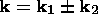(2)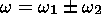(3)

In the case of two-dimensional interaction of three waves ( k,j), ( k1,j1), ( k2,j)2, from (2) follows [Volosevich et al., 1982]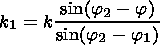(4)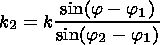(5)

If one takes into account the weak dispersion of FB waves, one finds in the case j=0 from (4) and (5) and the condition w=w1+w2 that(6)

The physical sense of the last relation lies in the fact that if two waves in the stage of linear generation with j=0 and j1<10o act via decay interaction, then the third wave must be in the region of linear damping with j2p/2.

Considering the system of equations consisting of the continuity equations of the particles, the MHD momentum balances and the Poisson equation for the electric field, neglecting in the electron momentum balance the inertial term, assuming quasineutrality ne=ni=n, and assuming potential electric field fluctuations with small amplitude E=-j, one has for the time derivatives of the high-frequency density variations [Volosevich and Meister, 2000a; Volosevich et al., 1982]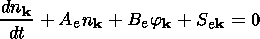(7)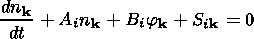(8)

Here nk=N/N0. n k and j k are the disturbances of the density of the charged particles and the electrostatic potential.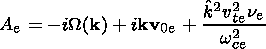(9)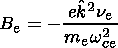(10)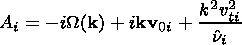(11)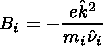(12)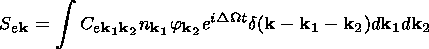(13)(14)(15)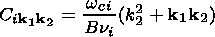(16)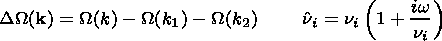(17)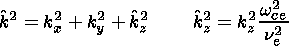(18)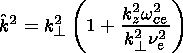(19)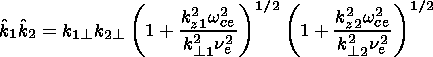(20)

W( k) is the frequency of the density variations with large time scales, d( k- k1- k2) is the delta function, and v0e and v0i are the electron and ion drift velocities that are determined by the mean electrostatic field E0. Se k and Si k are the coefficients of the nonlinear wave interaction of F-B waves. Within the linear theory, Se k and Si k equal zero. Then j k is proportional to n k. From (7) and (8) follows(21)

Further, substituting (21) into (13) and (14), the interaction coefficient of second order with respect to the nonlinear contributions S(2) k, k1, k2 is found, and so on. S(2) k, k1, k2 coincide with coefficients for decay processes given by Tsytovich [1970, 1971] and with coefficients occurring within the synchronism conditions of nonlinear optics. Results obtained in third order with respect to nonlinearity are presented in Volosevich and Meister [2000b].

Substituting (21) in (7) and (8) and taking S(2) and S(3) in (7) and (8) into account, the expressions for the amplitudes of the waves in third order with respect to the nonlinearity read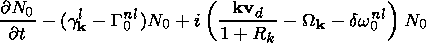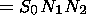(22)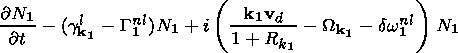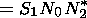(23)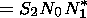(24)

Here, one has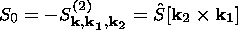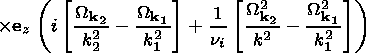(25)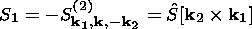(26)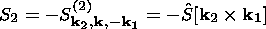(27)

where Nj=nkj/n0 are disturbances of the density of charged particles due to the waves number jj=0,1,2 ). gl kj and Gnlj designate the linear and nonlinear increments of the interacting waves,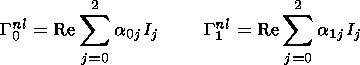(28)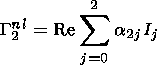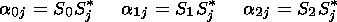(29)

and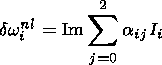(30)

is the nonlinear frequency shift, vd= v0e- v0i, Wkj is the frequency of the density variations,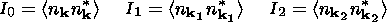(31)

The system (22)-(24) describes the interaction of four waves. In the relations (22)-(24), one sees the coefficients S(2) and not S(3), which describes the interaction of four waves (the moment of the fourth wave may be expressed by the momenta of the first three waves using the momentum balance of four coherent waves). Instead of S(3), Gnl k and dw0nl occur. Thus, the physical sense of the system (22)-(24) consists in the fact that, taking into account nonlinear effects of third order, the nonlinear wave interaction causes a nonlinear contribution to the growth rate and a nonlinear frequency shift. These phenomena result in the stabilization of the instability.

Further,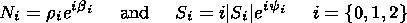(32)

are supposed, and the set of equations (22)-(24) is divided into real and imaginary expressions.

In the case of the low-frequency waves with wni, Re  SiIm  Si, the main effect of the wave interaction is the nonlinear phase shift. But for the high-frequency waves with w>ni and Re  SiIm  Si, nonlinear frequency shift and nonlinear contributions to the growth rate are of importance, and these nonlinear effects lead to the stabilization of the instability.

The system of equations (22)-(24) was solved numerically using the Runge-Kutta method and considering initial conditions near stable states. Applications were done for conditions with an unstable high-frequency wave (gl0>0), and two other linearly damped waves (gl1,gl2<0) ("large aspect angle" or "large flow angle").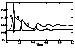Figure 1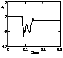Figure 2Figure 3
Examples of the numerical solution of (22)-(24) are presented in Figures 1, 2, 3, 4, and 5. Figure 1 shows the temporal evolution of the relative amplitudes r0, r1, r2 of the density waves with j=0;1;2 with respect to the background density for k=5 m-1 (in Figure 5, k=1 m -1 is considered). The time, t, is measured in seconds. In the initial state at t=0, the high-frequency wave j=0 is unstable and the low-frequency wave j=2 is damped as j2p/2. If r1 and r2 are small, the amplitude r0 grows exponentially. But with the time, if the amplitude r0 will be large enough, the amplitudes r1 and r2 also increase by nonlinear interaction. The amplitude of the linearly damped wave 2 and the relative phase of the waves q=b1+b2-b0 are shown in Figures 2 and 3, respectively. Nonlinear stabilization occurs if the time of the linear increase of the amplitude r0 of the unstable wave 1/gl0 is smaller than the time of the nonlinear interaction tnl. In the contrary case, the explosive instability may be excited.

From Figures 1, 2, and 3 follows, that under the condition that the initial relative amplitudes of the waves are small (about 10-3 ), then, for some time, the amplitude of the linearly unstable wave grows, and the amplitudes of the initially damped waves are small. But, if the amplitude of the unstable wave has grown up to a sufficient level, the nonlinear interaction results in an increase of the amplitudes of modes 1 and 2. This process leads to a quasi-stabilized state, the amplitudes of the interacting waves behave almost periodically, and the relative phase q approaches a constant value. When the quasi-periodic state is established, the amplitudes of the initially linearly damped waves are larger than the amplitude of the initially linearly growing wave. The stabilization of the waves at a finite amplitude is accomplished by modifying of the linear growth rate. It depends on the average wave fluctuation level and lasts until the nonlinear growth rate balances the linear growth rate.Figure 4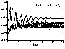Figure 5
The numerical simulations for large intervals of the parameters of the interacting waves showed that the solving the system of equations (22)-(24) depends on the wave parameters and the parameters of the ionosphere. The solution may be stationary, periodic, multi-periodic, quasi-stochastic, and stochastic. In Figure 4, results for the nonlinear interaction of waves with strong decay of the waves j=1, 2 are presented. In such a case, the solution is similar to a stochastic one [Vysshkind and Rabinovich, 1976; Wersinger et al., 1980]. It depends on the initial conditions and the relative phase q of the waves' increases slowly with the time.

### 3. Conclusions

The nonlinear interaction between high-frequency and low-frequency modes, excited by the FB instability in the collisional ionospheric E region during the linear stage of wave generation, is considered within the frame of MHD. Taking the MHD system of equation contributions of third order with respect to the nonlinearity into account, a self-consistent system of equations for the amplitudes and phases of three interacting waves is derived. The results of the numerical solution of the system of equations allows us to make the following conclusions:

1. If in the ionospheric plasma FB waves with linearly increasing amplitudes exist, then the most intensive wave interaction occurs in the propagation cone around the electron drift velocity with an angle of about jarccos v0e/csv0e is the electron drift velocity, cs represents the sound velocity). The wave interaction results in the generation of rather intensive waves that propagate perpendicularly to the electron drift velocity.

2. If the plasma system is in the weakly supercritical regime with electron drift velocities of the order of 1.1 sound velocities, the explosive instability may also occur. This means that, under such conditions, the interaction of four waves is not effective. With increasing level of supercriticality, the effectiveness of the three- and four-wave interactions grows.

3. If the case that the previously linear waves have wavelengths of the order of 1-5 m, the amplitudes of the waves beyond the linear region of evolution are of the order of 6% of the mean density, and the amplitudes of the nonlinear waves increase with increasing wavelength of the plasma disturbances and with growing electron drift velocity.

4. The nonlinear evolution of FB waves by coherent wave interaction can result in states of different types: quasi-stationary states for interacting low-frequency waves, periodic and multi-periodic behavior for high-frequency waves with w>ni (Figures 1, 2, and 3), and quasi-stochastic behavior (Figure 4). The evolution scenario depends on the plasma parameters ( ne, ni, Te, Ti, v0e ) and on the parameters of the interacting waves (the frequencies and growth rates as well as the ranges of the aspect and flow angles and the frequency of the unstable waves).

5. The results obtained may be used to interpret experimental data obtained during auroral radioscatter experiments.

#### Acknowledgments

A.V.V. thanks the organization Deutsche Forschungsgemeinschaft for financial support within the frame of contract WER 17/2/00 and the Fund of Fundamental Research of the Belarus Republic, project F-378. C.-V.M. gratefully thanks the Ministerium fur Wissenschaft, Forschung und Kultur des Landes Brandenburg for financial support by the HSP-3 project 24-04/055;2000.

### References

Fedorov, V. P., M. G. Gelberg, and A. V. Volosevich, A simulation of the evolution of low-frequency plasma turbulence in the ionospheric E region, in Ionospheric Response in the Solar Wind, edited by L. Triskova, Prague, 1988.

Gershman, B. N., L. M. Erukhimov, and Yu. Ya. Yashin, Wave Phenomena in the Ionosphere (in Russian), Nauka, Moscow, 1984.

Hamza, A. M., and J.-P. St.-Maurice, A self-consistent fully turbulent theory of auroral E region irregularities, J. Geophys. Res., 98, 11,601, 1993.

Hamza, A. M., and J.-P. St.-Maurice, Large aspect angles in auroral E region echoes. A self-consistent turbulent theory, J. Geophys. Res., 100 (A4), 5723, 1995.

Janhunen, P., Perpendicular particle simulation of the E region Farley-Buneman instability, J. Geophys. Res., 99 (A6), 11,461, 1994.

Lee, K., C. F. Kennel, and I. M. Kindel, High frequency Hall current instability, Radio Sci., 6, 209, 1971.

Liperovsky, V. A., C.-V. Meister, S. A. Senchenkov, K. V. Popov, M. A. Oleynik, and E. V. Liperovskaya, Consequences of current generation in the ionosphere caused by neutral wind action on Es clouds, Izv. Vuz. Radiofiz. (in Russian), 39 (2), 241, 1996.

Meister, C.-V., Farley-Buneman type waves caused by the influence of neutral winds on sporadic E layers, Abstr. Ser. German Astron. Soc., 11, 293, 1995.

Newman, A. L., and E. Ott, Nonlinear simulations of type 1 irregularities in the equatorial electrojet, J. Geophys. Res., 86, 6879, 1981.

Oppenheim, M., and N. Otani, Saturation of the Farley-Buneman instability via nonlinear EB drift instability, J. Geophys. Res., 101 (A8), 17,273, 1996.

Otani, N., and M. Oppenheim, A saturation mechanism for the Farley-Buneman instability, Geophys. Res. Lett., 35 (11), 1833, 1998.

Robinson, T. R., and F. Honary, A resonance broadening kinetic theory of the modified two-stream instability: Implications for radar auroral backscatter experiments, J.  Geophys. Res., 95, 1073, 1990.

Sahr, J. D., and B. G. Fejer, Auroral electrojet plasma irregularity theory and experiment: a critical review of present understanding and future directions, J. Geophys. Res., 101, 26,893, 1996.

Schlegel, K., and H. Thiemann, Particle-in-cell plasma simulations of the modified two-stream instability, Ann. Geophys., 12, 1091, 1994.

Sudan, R. N., Nonlinear theory of type I irregularities in the equatorial electrojet, Geophys. Res. Lett., 10, 983, 1983.

Tsytovich, V. N., Nonlinear Effects in Plasmas, Plenum Press, New-York, 1970.

Tsytovich, V. N., Theory of Turbulent Plasmas (in Russian), Gozatomizdat, Moscow, 1971.

Uspensky, M. V., et al., Auroral radar backscatter at off-perpendicular aspect-angles due to enhanced ionospheric refraction, J.  Geophys. Res., 99, 17,503, 1994.

Volosevich, A. V., Farley-Buneman instability in the polar ionosphere, in Phenomena in the Polar Ionosphere (in Russian), Nauka, Leningrad, 1978.

Volosevich, A. V., and Y. I. Galperin, Nonlinear wave structures in collisional plasma of auroral E region ionosphere, Ann. Geophys., 15, 899, 1997.

Volosevich, A. V., and V. A. Liperovsky, Generation of small-scale ingomogenities in a turbulent plasma and radio aurora, Geomagn. Aeron. (in Russian), 15 (1), 74, 1975

Volosevich, A. V., and C.-V.  Meister, Coherent three-wave interaction in the ionospheric plasma, Vestnik MG (in Russian), No. 6, edited by M. A. Avlasevich, Mogilev State University, Mogilev, Belarus, 2000a.

Volosevich, A. V., and C.-V. Meister, Nonlinear coherent four-wave interaction in space plasma, Astron. Nachr., 321, 263, 2000b.

Volosevich, A. V., V. A. Liperovsky, and M. A. Lifshits, Nonlinear mechanism of the stabilization of the Buneman-Farley instability, in Research of the High-Latitudinal Ionosphere and Magnetosphere (in Russian), Nauka, Leningrad, 1982.

Vysshkind, S. Y., and M. I. Rabinovich, The phase stochastization mechanism and structure of wave turbulence in dissipative media, Sov. Phys. JETP, 71 (218), 557, 1976.

Weiland, J., and H. Wilhelmsson, Coherent Nonlinear Interaction of Waves in Plasmas, Pergamon Press, New-York, 1977.

Wersinger, J.-M., J. M. Finn, and E. Ott, Bifurcation and "strange" behavior in instability saturation by nonlinear three-wave mode coupling, Phys. Fluids, 23 (3), 1142, 1980.

Load files for printing and local use. This document was generated by TeXWeb (Win32, v.1.3) on December 8, 2002.